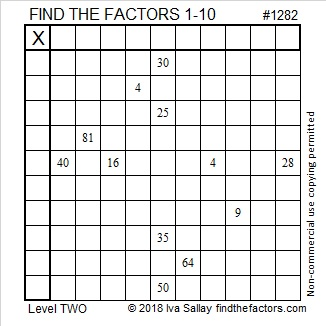# 1282 and Level 2

Can you find the factors from 1 to 10 that make the twelve clues in the puzzle the correct products for this scrambled multiplication table?Print the puzzles or type the solution in this excel file: 10-factors-1281-1288

Here is some information about the number 1282:

• 1282 is a composite number.
• Prime factorization: 1282 = 2 × 641
• The exponents in the prime factorization are 1 and 1. Adding one to each and multiplying we get (1 + 1)(1 + 1) = 2 × 2 = 4. Therefore 1282 has exactly 4 factors.
• Factors of 1282: 1, 2, 641, 1282
• Factor pairs: 1282 = 1 × 1282 or 2 × 641
• 1282 has no square factors that allow its square root to be simplified. √1282 ≈ 35.805031282 is the sum of two squares:
29² +  21² = 1282

1282 is the hypotenuse of a primitive Pythagorean triple:
400-1218-1282 calculated from 29² –  21², 2(29)( 21), 29² +  21²

The 21, 29, and 400 above are related to another Pythagorean triple:
20-21-29 because 20² = 400, 21² = 441 and 29² = 841. Thus,
400 + 441 = 841. Pretty cool!